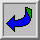- In the Old Testament, the 108th verse in Genesis reveals a major blessing as far as it is about the creation of man and woman :

" [... ] Male and female created he them; and blessed them, and called their name Adam, in the day when they were created. (Gen 5/2).

- In the New Testament, the 108th verse in the Matthew's gospel is of a major importance with a notion of achieved plenitude and complete fulfillment of the Holy Scriptures :

" [... ] For verily I say unto you, Till heaven and earth pass, one jot or one tittle shall in no wise pass from the law, till all be fulfilled. (Mt 5/18).

- It is in this verse that appears the only occurrence of the word " jot ".

To continue with some symbolic values often used in esoterism:

- The earth cycle is supposed to be of 2160 years = 20 x 108

- The Buddhist rosary, where from is inspired the rosary of the Moslems, then straight-away as an inheritance of crusades by (" Our lady worshiping ") Catholics, is constituted of 108 fragments of distinctive different human skulls .

- 108 sacred books constitute the holy Writings for Tibetans.

- According to the Chinese tradition, it is necessary to walk for " 108000 LI " to reach wisdom and:

108 000 x " LI " = 108 000 x 576 m = 62208 km

- In French or English, (if A = 1, B = 2), the alphanumeric addition of the letters of the word " kilometre " or " kilometer " gives 108

- It is also a revealing principle because, as an example and according to the Judeo - Christian calendar, if 108 + 151 = 259, the 259th day of a leap year is also in the [-108] day.

Important remark:

sign (-) is not at all " negative " in that specific case of figure . It is only a calendar position related to a countdown.

- The last ostension of the Holy Shroud was at first forecast from April 18 to May 31, 1998, which are respectively the 108th and 151-th day of the year. It was prolonged until June 14, 1998.

- A leap year displays 366 days and 3 x 6 x 6 give 108.

Considering that the number 108 corresponds also to the following sequences:

2 + 2 + 2

or

36 + 36 + 36

the 108th hour of February falls in the middle of the 36th day.

The tenth of August (every year) corresponding to " 10.8 " (according to the French datation system), stands at 144 days from the end of any (leap or not) year .

- The number 108 amounts to 3/4 of the number 144

144 000 - 144 = [108 + 108] x 666

144 000 : [108 + 108] = 666 + 144

and as far as 108 = 2 + 2 + 2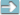144 000 - 144 = [108 + 108] x 666144 000 : [108 + 108] = 666 + 144144 000 - 144 = [2 + 2 + 2] + [2 + 2 + 2] x 666144 000 : [2 + 2 + 2] + [2 + 2 + 2] = 666 + 144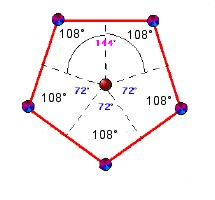Heart of a Pentagone

Five 108° angles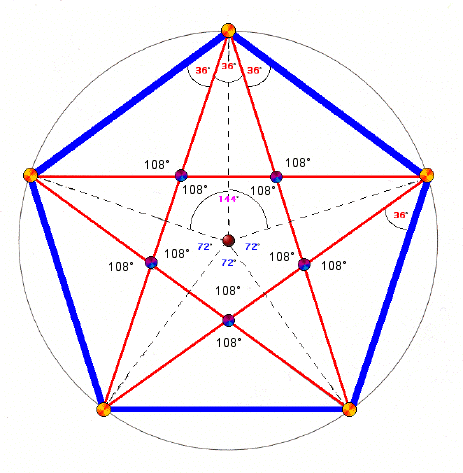Pentagones...

From a more general point of view:

- The frequency of radioelectric waves used for broadcasting on the FM band is set between 88 and 108 MHz.

- The Vedanta, according to the Hinduist tradition, recognizes 108 authentic doctrines (Upanishad) aiming to approach the Truth and to destroy Ignorance.

These few remarks about the number 108 seem totally unstitched. They allow however to highlight this number as a major principle of revelation which enables to establish the " positive " or " negative " character of certain " hidden " links or considered as to be so.

As a consequence, this number is quite unavoidable to study and understand those that are treated during the following chapters.

Table of the first hundred multiples of number 108:

 108 x 1 = 108 108 x 35 = 3780 108 x 69 = 7452 108 x 2 = 216 108 x 36 = 3888 108 x 70 = 7560 108 x 3 = 324 108 x 37 = 3996 108 x 71 = 7668 108 x 4 = 432 108 x 38 = 4104 108 x 72 = 7776 108 x 5 = 540 108 x 39 = 4212 108 x 73 = 7884 108 x 6 = 648 108 x 40 = 4320 108 x 74 = 7992 108 x 7 = 756 108 x 41 = 4428 108 x 75 = 8100 108 x 8 = 864 108 x 42 = 4536 108 x 76 = 8208 108 x 9 = 972 108 x 43 = 4644 108 x 77 = 8316 108 x 10 = 1080 108 x 44 = 4752 108 x 78 = 8424 108 x 11 = 1188 108 x 45 = 4860 108 x 79 = 8532 108 x 12 = 1296 108 x 46 = 4968 108 x 80 = 8640 108 x 13 = 1404 108 x 47 = 5076 108 x 81 = 8748 108 x 14 = 1512 108 x 48 = 5184 108 x 82 = 8856 108 x 15 = 1620 108 x 49 = 5292 108 x 83 = 8964 108 x 16 = 1728 108 x 50 = 5400 108 x 84 = 9072 108 x 17 = 1836 108 x 51 = 5508 108 x 85 = 9180 108 x 18 = 1944 108 x 52 = 5616 108 x 86 = 9288 108 x 19 = 2052 108 x 53 = 5724 108 x 87 = 9396 108 x 20 = 2160 108 x 54 = 5832 108 x 88 = 9504 108 x 21 = 2268 108 x 55 = 5940 108 x 89 = 9612 108 x 22 = 2376 108 x 56 = 6048 108 x 90 = 9720 108 x 23 = 2484 108 x 57 = 6156 108 x 91 = 9828 108 x 24 = 2592 108 x 58 = 6264 108 x 92 = 9936 108 x 25 = 2700 108 x 59 = 6372 108 x 93 = 10044 108 x 26 = 2808 108 x 60 = 6480 108 x 94 = 10152 108 x 27 = 2916 108 x 61 = 6588 108 x 95 = 10260 108 x 28 = 3024 108 x 62 = 6696 108 x 96 = 10368 108 x 29 = 3132 108 x 63 = 6804 108 x 97 = 10476 108 x 30 = 3240 108 x 64 = 6912 108 x 98 = 10584 108 x 31 = 3348 108 x 65 = 7020 108 x 99 = 10692 108 x 32 = 3456 108 x 66 = 7128 108 x 100 = 10800 108 x 33 = 3564 108 x 67 = 7236 ... 108 x 34 = 3672 108 x 68 = 7344

! Warning: the use or any particular attention given to figures or to numbers (considered as lucky ones or signs of misfortune) reveals superstitious beliefs or convictions in touch with magical practice and are strictly condemned in the Holy Scriptures.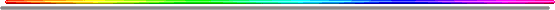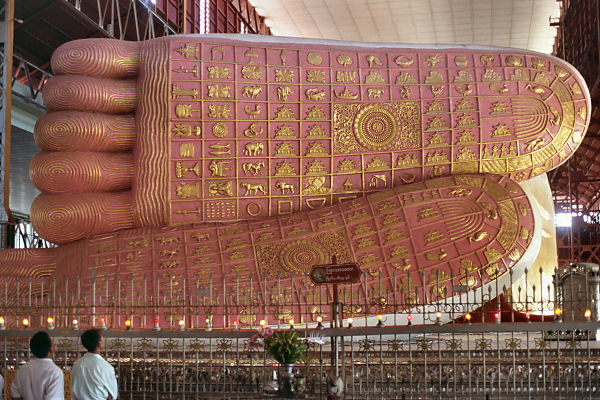108 symbols can be spotted on each foot of this BuddhaBible and Numbers End Times Interpreted with the Help of the Bible, Numbers, and the French Language, by Anne E. Crews and Gérard Colombat (author of this website) "Was 9/11 supposed to happen, and is it related to the Rapture of the Christian Church? If so, how? Are all world events based on a coded numerical system, and if so, to whom does the coded numerical system point…the MESSIAH Jesus Christ? Bible and Numbers is a factual attempt to answer these lingering questions. Based on over thirty years of research by author Gérard Colombat, authors Anne E. Crews and Colombat proffer an intelligent and insightful theory in their quest for biblical truth. By juxtaposing a numerical system based on the modern calendar, sacred Bible numbers, and the phonetics and numerics of the French language, Colombat and Crews seek to define the occurrences of past events in conjunction with the present, offering that through the Bible and Numbers, questions of the future can be answered today. " 340 pages - \$18.99 (paperback) http://www.tatepublishing.com/bookstore/book.php?w=978-1-60462-558-5